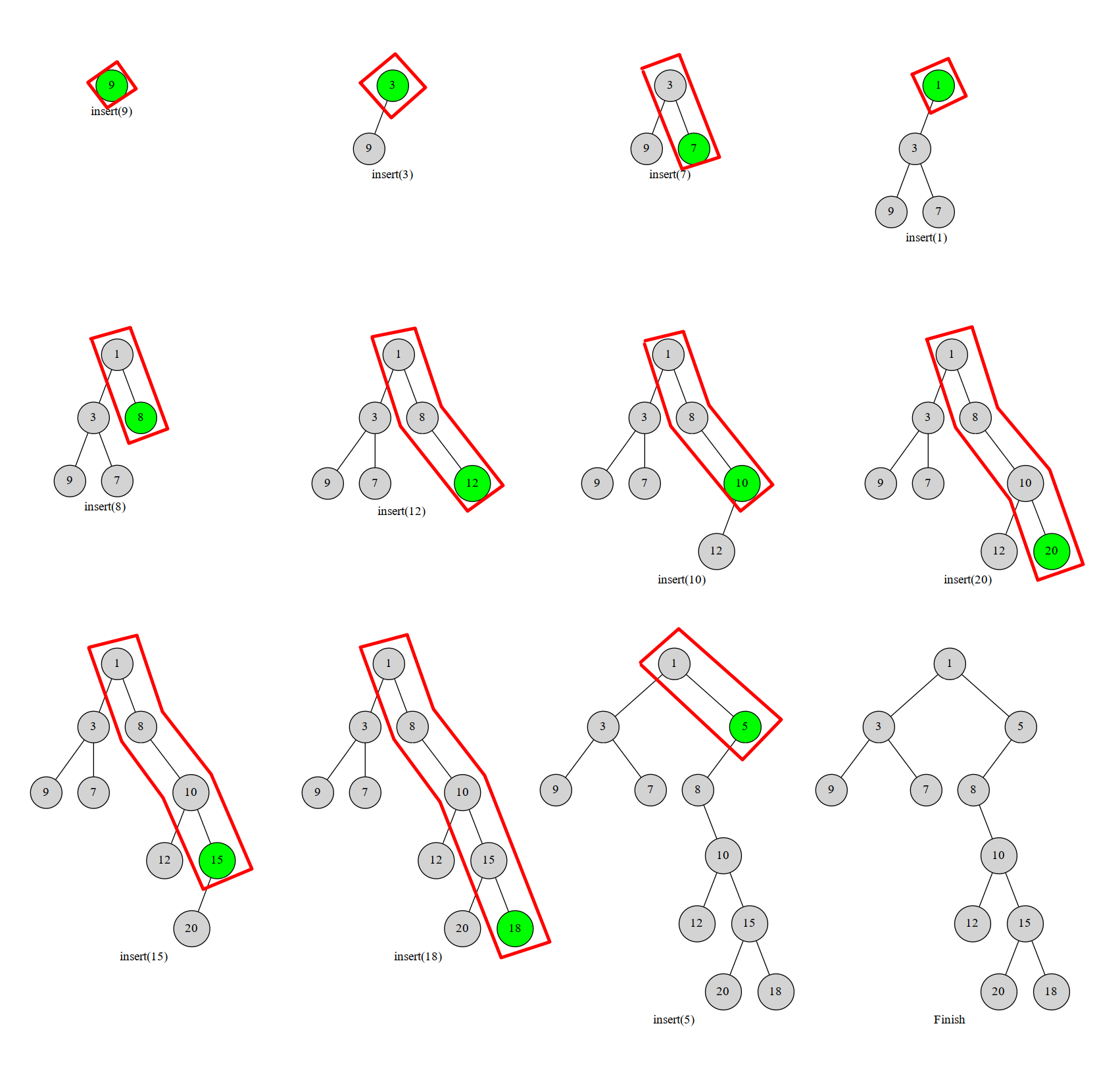# 笛卡尔树（图源自维基百科）

## 构建¶

### 栈构建¶1 2 3 4 5 6 7 8 9 10 11 新建一个大小为 n 的空栈。用 top 来标操作前的栈顶，k 来标记当前栈顶。 For i := 1 to n k := top While 栈非空 且 栈顶元素 > 当前元素 k-- if 栈非空 栈顶元素.右儿子 := 当前元素 if k < top 当前元素.左儿子 := 栈顶元素 当前元素入栈 top := k 
 1 2 3 4 5 6 7 8 for (int i = 1; i <= n; i++) { int k = top; while (k > 0 && h[stk[k]] > h[i]) k--; if (k) rs[stk[k]] = i; // rs代表笛卡尔树每个节点的右儿子 if (k < top) ls[i] = stk[k + 1]; // ls代表笛卡尔树每个节点的左儿子 stk[++k] = i; top = k; } 

## 例题¶

HDU 1506 最大子矩形1 2 3 4 5 6 7 8 9 10 11 12 13 14 15 16 17 18 19 20 21 22 23 24 25 26 27 28 29 30 31 32 33 34 35 36 37 38 39 40 41 42 43 44 45 46 47 48 49 50 51 52 53 54 55 56 #include #include #include #include using namespace std; typedef long long ll; const int N = 100000 + 10, INF = 0x3f3f3f3f; struct node { int idx, val, par, ch; friend bool operator<(node a, node b) { return a.idx < b.idx; } void init(int _idx, int _val, int _par) { idx = _idx, val = _val, par = _par, ch = ch = 0; } } tree[N]; int root, top, stk[N]; ll ans; int cartesian_build(int n) { // 建树，满足小根堆性质 for (int i = 1; i <= n; i++) { int k = i - 1; while (tree[k].val > tree[i].val) k = tree[k].par; tree[i].ch = tree[k].ch; tree[k].ch = i; tree[i].par = k; tree[tree[i].ch].par = i; } return tree.ch; } int dfs(int x) { // 一次dfs更新答案就可以了 if (!x) return 0; int sz = dfs(tree[x].ch); sz += dfs(tree[x].ch); ans = max(ans, (ll)(sz + 1) * tree[x].val); return sz + 1; } int main() { int n, hi; while (scanf("%d", &n), n) { tree.init(0, 0, 0); for (int i = 1; i <= n; i++) { scanf("%d", &hi); tree[i].init(i, hi, 0); } root = cartesian_build(n); ans = 0; dfs(root); printf("%lld\n", ans); } return 0; }